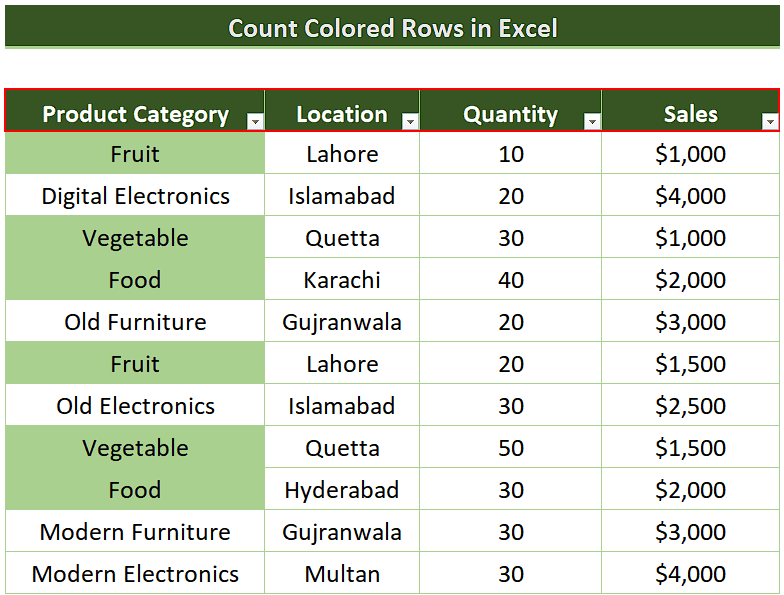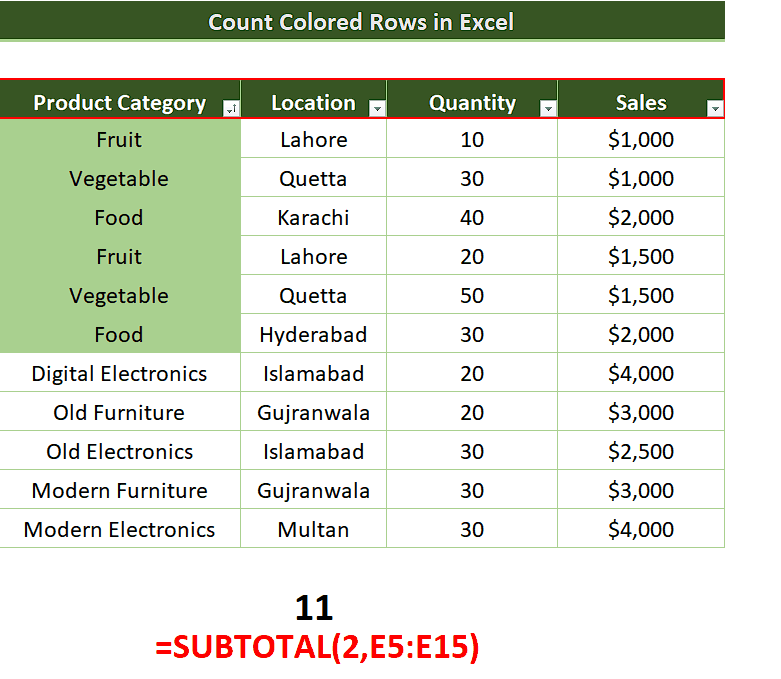# How to count filtered rows in Excel

In this tutorial, we are going to learn how to Count Filtered Rows by the above mentioned method in Excel. Let’s take a look at the following sales dataset.

## Use Filter and SUBTOTAL

In this method we’ll apply a specific filter on the data range to filter the data using the color filled in the cell and then use SUBTOTAL function to count the rows only that are colored. Follow along the steps explained below to achieve this goal.Microsoft Excel is one of the best tools which provides many built-in tools and features that can help the data analysts in visualizing data in the best possible ways. One such feature is the ability of Excel to apply filters on datasets to temporarily hide the unwanted information. However, sometimes after applying the filter on the data we need to gather information about the filtered data only, such as counting the number of data points in a filtered data. We can get it done by using the following method;

Apply Filter and then use SUBTOTAL

### Step 1 – Apply the filter using shortcut key CTRL+SHIFT+L– Select a cell inside your dataset.
– Use the shortcut key to apply a filter to the dataset by pressing CTRL+SHIFT+L.
– This will apply the filter to all columns in the data range automatically.
– The same thing can also be done by going to the Sort & Filter option in the Editing group on the Home tab from the list of main tabs in Excel.

Now that we have applied the filter to our data, we can use it as per our requirements to see only the data that we are interested in. Let’s assume that we wish to see the sales data of eatables which are colored green in the dataset such as Fruit, Vegetable and Food items only from the Product Category column. So, let’s use the filter options to get it done.

### Step 2 – Use Filter by Color to filter rows in Excel– We’ll go to the column header of interest, which in our case is the Product Category and click on the dropdown arrow.
– This will open up new options and we’ll click the Filter by Color option this will give us the option to choose between all the cell colors available in our data range. Since we have only one color i.e. (green)   therefore, we’ll see this color and No fill option.
– We’ll choose the (green) option and all our data will be filtered and we’ll see only the green colored cells.

### Step 3 – Use SUBTOTAL function to count the filtered cells– Now we’ll use the subtotal function to count the number of rows as a result of application of the color filter. We’ll use the following simple formula;
=SUBTOTAL(2,E5:E15)
– In the above formula, we are counting the colored cells indirectly by counting the sub rows that contain numeric data. This will give us the count of the rows only which are colored.

This is how you can count the number of filtered rows in Excel.